## Tables index

#### Abdallah S. A. Yaseen

American Journal of Applied Mathematics and Statistics. 2022, 10(1), 28-38 doi:10.12691/ajams-10-1-5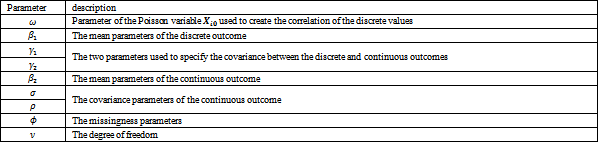• Table 1. Parameter description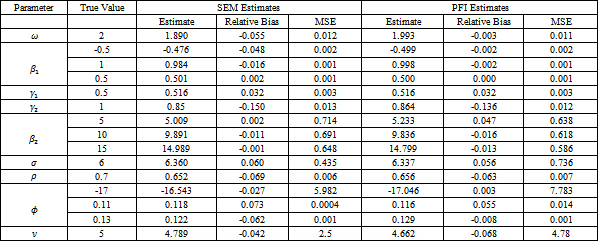• Table 2. Parameter estimates, relative bias and mean square error of the SEM and PFI estimates, degree of freedom ν is unknown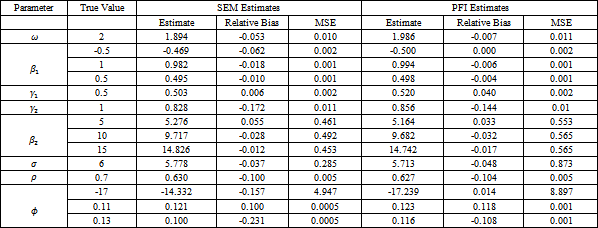• Table 3. Parameter estimates, relative bias and mean square error of the SEM and PFI estimates, degree of freedom ν is known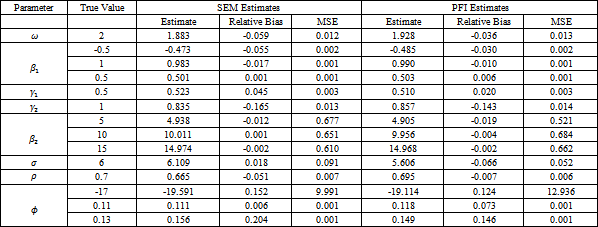• Table 4. Parameter estimates, relative bias and mean square error of the SEM and PFI estimates, degree of freedom ν is fixed at 5, true distribution is multivariate normal distribution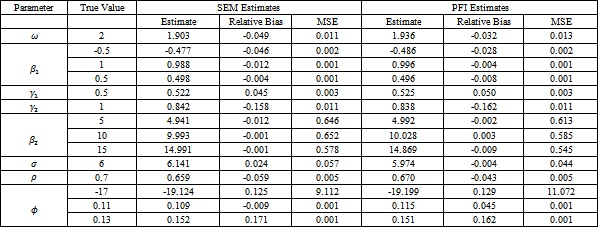• Table 5. Parameter estimates, relative bias and mean square error of the SEM and PFI estimates, degree of freedom ν is fixed at 15, true distribution is multivariate normal distribution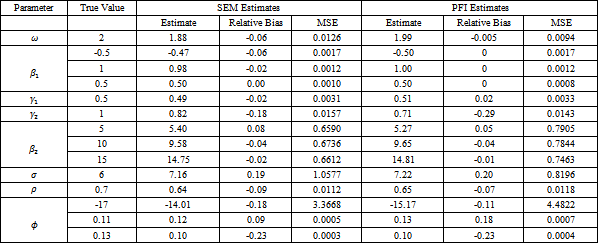• Table 6. Parameter estimates, relative bias and mean square error of the SEM and PFI estimates, true distribution is multivariate t distribution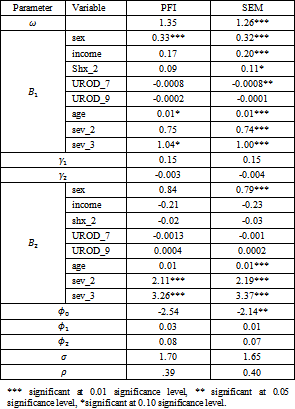• Table 7. Parameter estimates of the ICDB data and its significance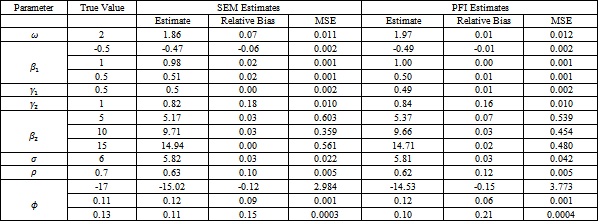• Table A-1. Parameter estimates, relative bias and mean square error of the SEM and PFI estimates, Yaseen and Gad (2019) results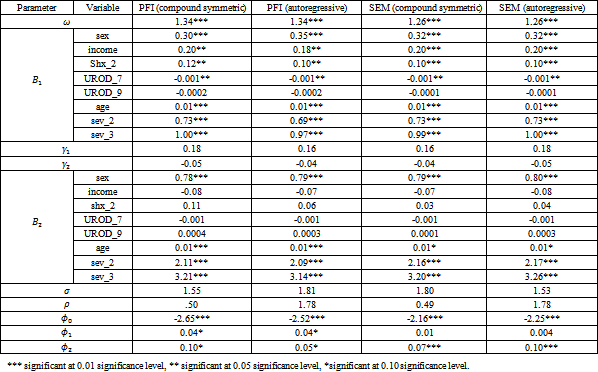• Table A-2. Parameter estimates of the ICDB data and its significance under normality assumption, Yaseen and Gad (2019) results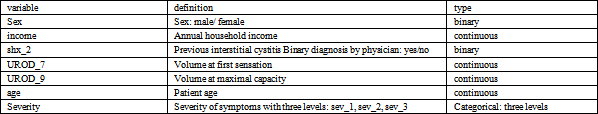• Table A-3. The definition of the covariates used in ICDB data